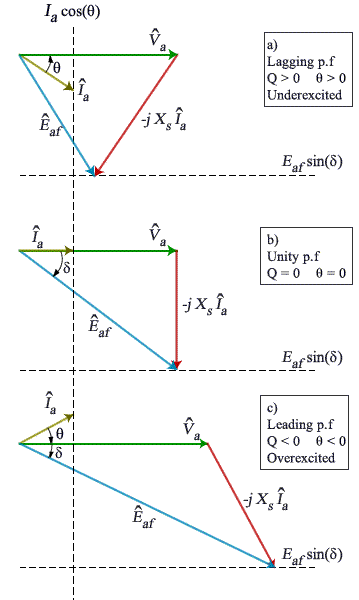ECE 449 - Power Systems Laboratory

# Experiment 7: Synchronous Motor V-Curve

## Objectives

1. To practice designing an experiment
2. To obtain the no-load V-curve of a synchronous motor

## Theory

Unloaded synchronous motors are used in parallel with the receiving end of a transmission line to improve line voltage regulation. The motor, with proper field excitation, can act like capacitor on its input side and thus draw leading current to compensate for the lagging current taken by an inductive load.  A V-curve of a synchronous motor shows the relation between armature currents as ordinates and field currents as abscissas when the motor operates with a constant power input at rated terminal voltage.  A family of V-curves may be obtained for a given motor by observing the above relations for a number of values of constant power inputs.

The input power to a three-phase synchronous motor is given by  , and if P is kept constant and V is naturally constant because the motor is connected to the power supply, it follows that for any given V-curve if I varies, cos φ must also change.  The minimum point of the V-curve represent the condition of unity power factor (pf).  The motor appears as inductive load if the field current is less than that corresponding to the minimum point on the V-curve; and it appears as capacitive load for field currents above that.

The equivalent circuit per phase is shown in the Fig 1 with the armature resistance neglected.  Êaf is the induced back emf due to the flux created by the rotor current If.  If saturation is neglected then Êaf   is proportional to If Va is the applied voltage per phase and usually taken as a reference.  The angle of Êaf   with Va is the power angle δ.  Another expression of the power given to the motor is: P = 3(Va Êaf  / X ) sin(δ ).  Fig 2 shows that when Êaf   increases (by increasing the field current) and with constant power, the negative power factor angle increases (less negative) then it becomes zero, and with further increase in the field current the angle becomes positive, i.e., the motor is consuming negative Q (supplying Q to the grid). ÊFig. 2. Phasor diagrams for different excitations and constant power of a synchronous motor.

From the above figure and from what you learned in the energy conversion course, it should be clear that for a constant P given to the motor, the armature current depends on the field current.  When the motor consumes reactive power, the power factor is lagging and the armature current is large (Fig 2.a).  As the field current increases, the power factor improves, the pf angle becomes smaller (but still negative), and the armature current decreases.  For a certain field current, the pf angle is zero and the armature current is smallest for a certain P.  Increasing the field current further, would make the pf leading, the motor will supply Q and the armature current would start increasing again.

The objective of this experiment is to obtain a graph of the armature current (y-axis) against the field current (x-axis).

## Procedure

1. Using the 10-hp motor-generator set (DC-AC) that you used in the Alternator Synchronization experiment, design an experiment to obtain the V-curve for a lightly loaded synchronous machine.
2. Your design should include a diagram to measure the three-phase power supplied to the AC motor using the available connecters and equipments.
3. Drive a dc generator with a wye-connected synchronous motor. Arrange to measure watts, volts and amperes at the motor input, the field current of the motor, and V and I at the DC generator output.
4. Observe the ratings of the motor, the DC generator, and the load, then decide on a few input power levels to which you will obtain the V-curves.
5. Change the field current of the synchronous motor and measure If   and Ia.  Observe when Ia becomes minimum and take a few readings above and below this minimum value.
6. Switch off the supply to the motor and connect a resistive load to the DC generator.
7. Change the power input to the synchronous motor by changing the resistive load and/or the field rheostat of the field circuit of the DC generator.
8. For each load, measure the input power, the input current, the field current of the motor, the output DC current and voltage.

## Circuit Diagram

Draw detailed circuit diagrams.

## Report

1. Plot line current vs field current for the synchronous motor for the no-load case.
2. What happens to the V- Curve when a synchronous motor is loaded?
3. Explain how this machine can be used to correct the power factor of a 3phase lagging load.
4. Discuss methods of starting 3-phase synchronous motors.
5. Show by phasors why the power factor at the input to a synchronous motor changes with field current.
6. It is desired to install a synchronous motor to improve the power factor of an induction motor load of 1500 kilowatts with an average power factor of 0.8 lagging. If the synchronous motor is to replace a portion of the induction motor load and is to operate at 0.7 power factor leading, what is the kva of synchronous motor required to bring the power factor of the total load to 0.9 lagging? What kva induction motors is replaced by the synchronous motor if the replaced induction motors have an average power factor of 0.7 lagging?